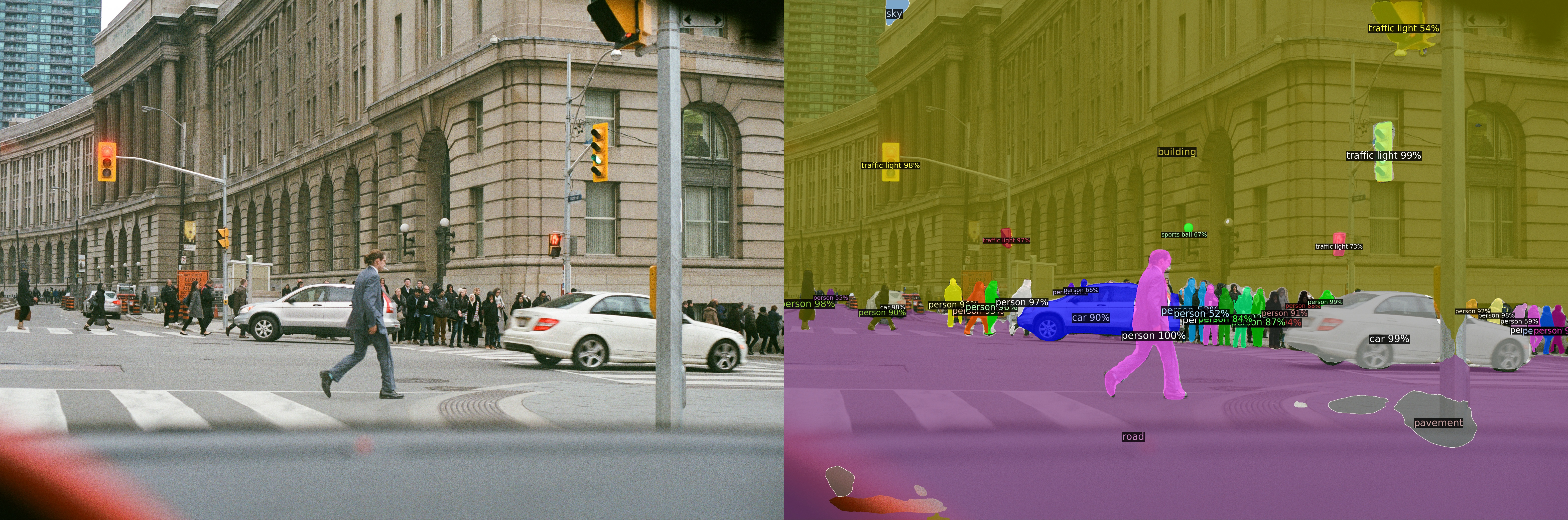# Introduction to Panoptic Segmentation: A Tutorial

Friday, October 18, 2019

In semantic segmentation, the goal is to classify each pixel into the given classes. In instance segmentation, we care about segmentation of the instances of objects separately. The panoptic segmentation combines semantic and instance segmentation such that all pixels are assigned a class label and all object instances are uniquely segmented.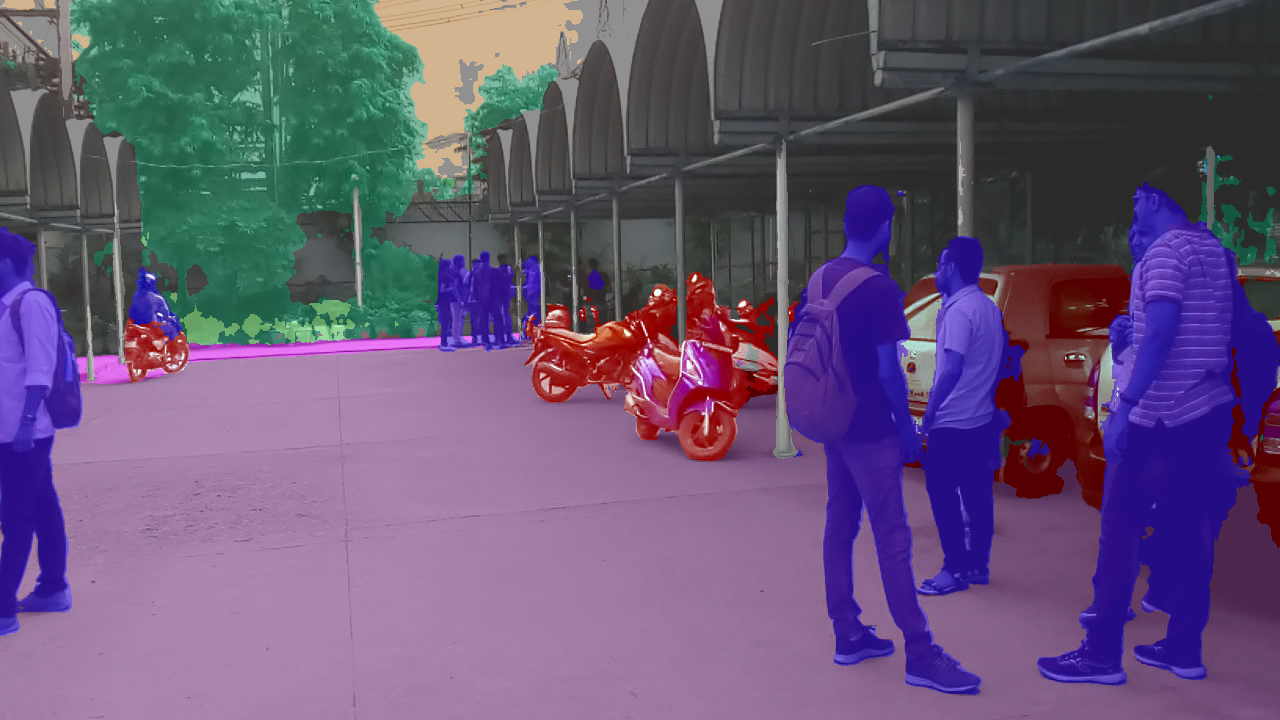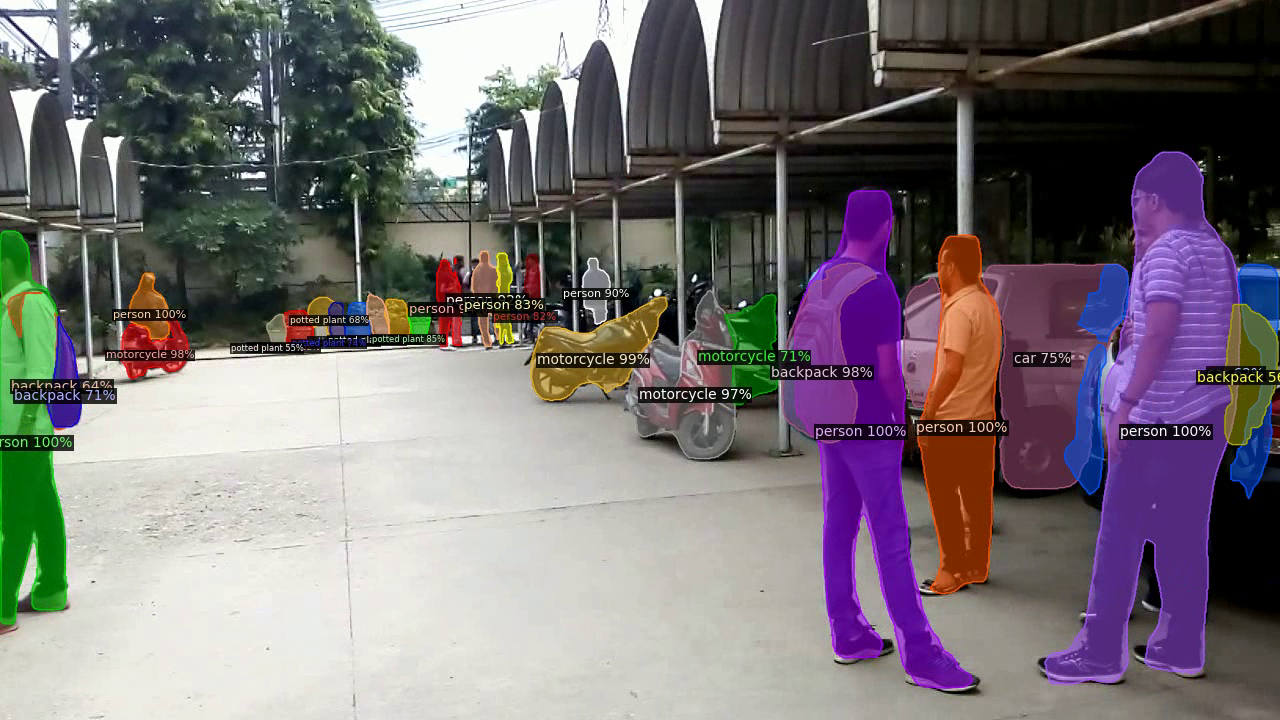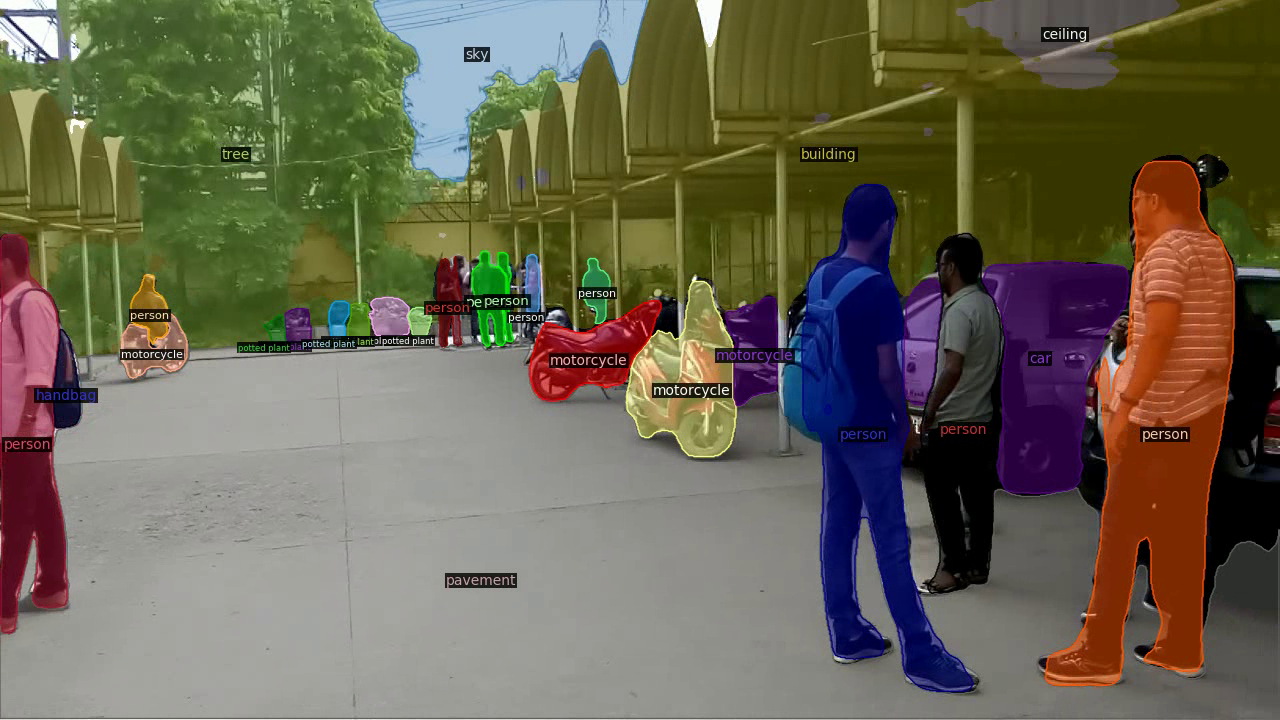## Introduction

The goal in panoptic segmentation is to perform a unified segmentation task. In order to do so, let’s first understand few basic concepts.

A thing is a countable object such as people, car, etc, thus it’s a category having instance-level annotation. The stuff is amorphous region of similar texture such as road, sky, etc, thus it’s a category without instance-level annotation. Studying thing comes under object detection and instance segmentation, while studying stuff comes under semantic segmentation.

The label encoding of pixels in panoptic segmentation involves assigning each pixel of an image two labels – one for semantic label, and other for instance id. The pixels having the same label are considered belonging to the same class, and instance id for stuff is ignored. Unlike instance segmentation, each pixel in panoptic segmentation has only one label corresponding to instance i.e. there are no overlapping instances.

For example, consider the following set of pixel values in a naive encoding manner:

26000, 260001, 260002, 260003, 19, 18


Here, pixel // 1000 gives the semantic label, and pixel % 1000 gives the instance id. Thus, the pixels 26000, 26001, 260002, 26003 corresponds to the same object and represents different instances. And, the pixels 19, and 18 represents the semantic labels belonging to the non-instance stuff classes.

In COCO, the panoptic annotations are stored in the following way:

Each annotation struct is a per-image annotation rather than a per-object annotation. Each per-image annotation has two parts: (1) a PNG that stores the class-agnostic image segmentation and (2) a JSON struct that stores the semantic information for each image segment.

## Datasets

The available panoptic segmentation datasets include MS-COCO, Cityscapes, Mapillary Vistas, ADE20k, and Indian Driving Dataset.

## Evaluation

In semantic segmentation, IoU and per-pixel accuracy is used as a evaluation criterion. In instance segmentation, average precision over different IoU thresholds is used for evaluation. For panoptic segmentation, a combination of IoU and AP can be used, but it causes asymmetry for classes with or without instance-level annotations. That is why, a new metric that treats all the categories equally, called Panoptic Quality (PQ), is used.

As in the calculation of AP, PQ is also first calculated independently for each class, then averaged over all classes. It involves two steps: matching, and calculation.

Step 1 (matching): The predicted and ground truth segments are considered to be matched if their IoU > 0.5. It, with non-overlapping instances property, results in a unique matching i.e. there can be at most one predicted segment corresponding to a ground truth segment.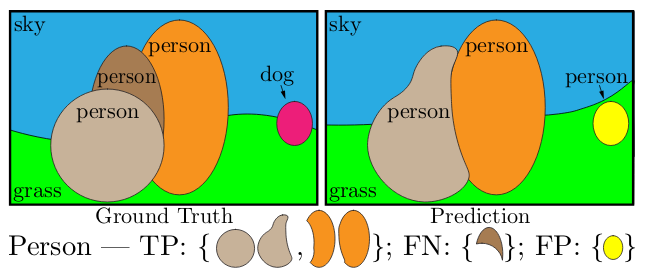Step 2 (calculation): Mathematically, for a ground truth segment g, and for predicted segment p, PQ is calculated as follows.

\begin{align} \mathrm{PQ} &= \frac{\sum_{(p, g) \in T P} \operatorname{IoU}(p, g)}{|T P|+\frac{1}{2}|F P|+\frac{1}{2}|F N|}\\ &= \underbrace{\frac{\sum_{(p, g) \in T P} \operatorname{loU}(p, g)}{|T P|}}_{\text {segmentation quality (SQ) }} \times \underbrace{\frac{|T P|}{|T P|+\frac{1}{2}|F P|+\frac{1}{2}|F N|}}_{\text {recognition quality (RQ) }} \end{align}

Here, in the first equation, the numerator divided by TP is simply the average IoU of matched segments, and FP and FN are added to penalize the non-matched segments. As shown in the second equation, PQ can divided into segmentation quality (SQ), and recognition quality (RQ). SQ, here, is the average IoU of matched segments, and RQ is the F1 score.

## Model

One of the ways to solve the problem of panoptic segmentation is to combine the predictions from semantic and instance segmentation models, e.g. Fully Convolutional Network (FCN) and Mask R-CNN, to get panoptic predictions. In order to do so, the overlapping instance predictions are first need to be converted to non-overlapping ones using a NMS-like (Non-max suppression) procedure.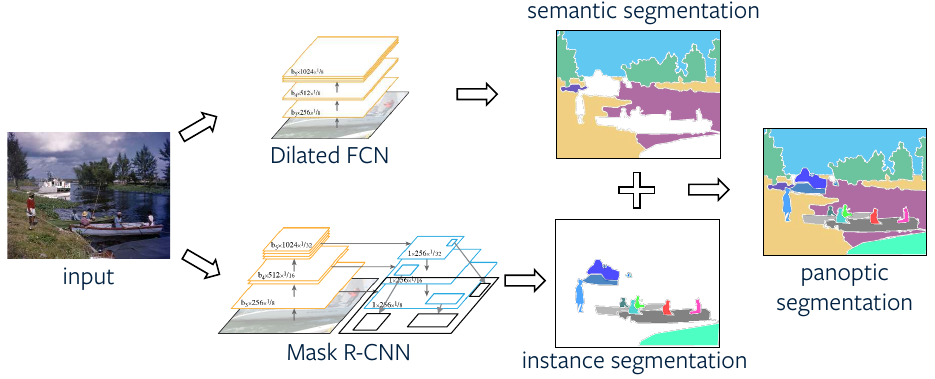A better way is to use a unified Panoptic FPN (Feature Pyramid Network) framework. The idea is to use FPN for multi-level feature extraction as backbone, which is to be used for region-based instance segmentation as in case of Mask R-CNN, and add a parallel dense-prediction branch on top of same FPN features to perform semantic segmentation.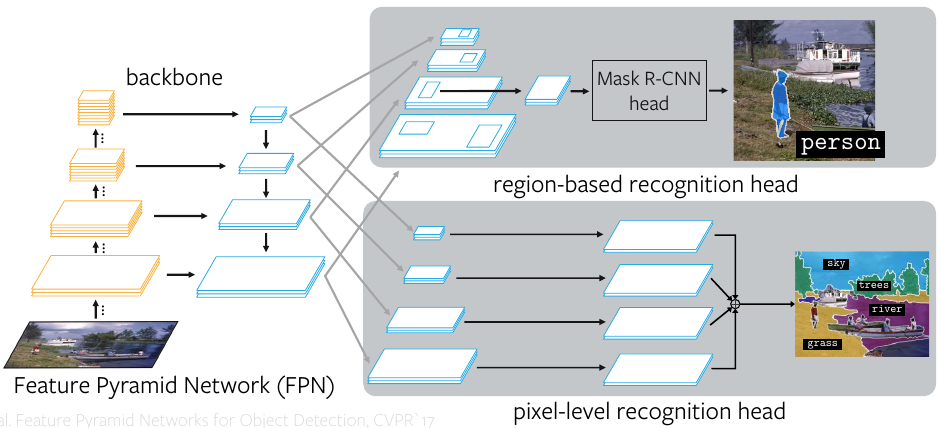During training, the instance segmentation branch has three losses $$L_{cls}$$ (classification loss), $$L_{bbox}$$ (bounding-box loss), and $$L_{mask}$$ (mask loss). The semantic segmentation branch has semantic loss, $$L_s$$, computed as the per-pixel cross-entropy between the predicted and the ground truth labels.

$L = \lambda_i(L_{cls} + L_{bbox} + L_{mask}) + \lambda_s L_s$

In addition, a weighted combination of the semantic and instance loss is used by adding two tuning parameters $$\lambda_i$$ and $$\lambda_s$$ to get the panoptic loss.

## Implementation

Facebook AI Research recently released Detectron2 written in PyTorch. In order to test panoptic segmentation using Mask R-CNN FPN, follow the below steps.